# The Basics of Functions

Definition. A function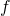from a set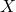to a set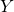is a relation which satisfies the following two conditions:

1.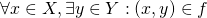, and
2.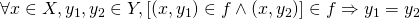That is, a function assigns to each (clause 1) element ofan element of, and that assignment is unambiguous (clause 2). Since we could rewrite clause 1 as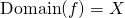(why?), we'll refer to clause 1 as the "domain clause" and clause 2 as the "unambiguousness clause".

Notation. Instead of the mouthfulis a function fromto, we write the shorthand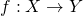.

We also have this familiar notation:

Notation. Ifis a function fromto, and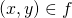, we write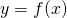.

Observe that if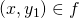and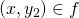, then this notation reads as follows: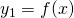and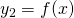, so we'd darn well better have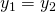, or else something is very wrong.

There is another way to represent a function, by specifying its domain and its rule:

Notation. Ifis a function fromto, we write. To specify the rule thatuses, we write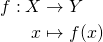For example, we could denote the function which takes in a real number as input, and squares it by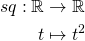Notice that the arrow between the sets is a different shape from the one between elements of the set. As usual we want to let go of our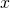s, so we could as well have written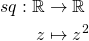or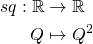This frees us from the tyranny of always writing.